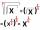Solve pure quadratic equation.

Result

x1 =  0.55
x1 =  -0.55

Solution:Leave us a comment of example and its solution (i.e. if it is still somewhat unclear...):Be the first to comment!To solve this example are needed these knowledge from mathematics:

Looking for help with calculating roots of a quadratic equation? Do you have a linear equation or system of equations and looking for its solution? Or do you have quadratic equation?

Next similar examples:Solve quadratic equation: 2x2-58x+396=0
2. EQ2Solve quadratic equation: ?
3. Solve 3Solve quadratic equation: (6n+1) (4n-1) = 3n2
4. ProductThe product of two consecutive odd numbers is 8463. What are this numbers?Quadratic equation ? has roots x1 = 80 and x2 = 78. Calculate the coefficients b and c.
6. EquationEquation ? has one root x1 = 8. Determine the coefficient b and the second root x2.Find the roots of the quadratic equation: 3x2-4x + (-4) = 0.
8. FractionFor what x expression ? equals zero?
9. DiscriminantDetermine the discriminant of the equation: ?
10. Square rootsWhat is equal to the product of the square roots of 295936?
11. EquationSolve the equation: 1/2-2/8 = 1/10; Write the result as a decimal number.
12. Simple equationSolve for x: 3(x + 2) = x - 18
13. Fifth of the numberThe fifth of the number is by 24 less than that number. What is the number?
14. CandyPeter had a sachet of candy. He wanted to share with his friends. If he gave them 30 candies, he would have 62 candies. If he gave them 40 candies, he would miss 8 candies. How many friends did Peter have?
15. Unknown numberIdentify unknown number which 1/5 is 40 greater than one tenth of that number.
16. CalculationHow much is sum of square root of six and the square root of 225?
17. Theorem proveWe want to prove the sentence: If the natural number n is divisible by six, then n is divisible by three. From what assumption we started?